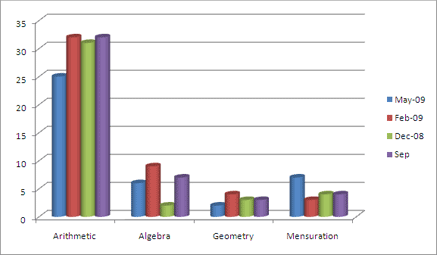# Quantitative AptitudeMAT Exam
Explore more-MAT
KI Unique
Test Yourself
Help

## QUANTITATIVE APTITUDE

This section tests the mathematical skills of the examinee. It contains 40 questions and carries 40 marks. The time allotted for this section is 40 minutes.
It covers various topics of algebra, arithmetic, geometry and area mensuration.

### ARITHMETIC

• Number system and theory
• Percentages
• Profit & Loss
• Speed, time & distance
• Pipes & Cisterns
• Races
• Averages
• Mixtures & Allegations
• Ratio & proportion

### ALGEBRA

• Linear & Quadratic Equations
• Logarithm
• Progressions
• Inequalities
• Probability
• Permutation & Combination
• Function
• Set Theory

### GEOMETRY

• Geometry
• Co-ordinate Geometry
• Trignometry

### AREA & MENSURATION

• Area & Perimeter
• Volume
• Quadilaterals,Parllellograms and Polygons
• Circles
The weightage of each sort of questions can be calculated by comparing the analysis of recent Mat exams.

### MAT QUANTITATIVE APTITUDE ANALYSIS:This analysis shows that arithmetic topic covers the maximum percentage of sums in MAT. However other question based on reasoning in MAT can come in the form of puzzles in Quantitative section. This section can turn out to be the most scoring section. Analysis of MAT recent papers proved that it is a scoring section and questions in it are either easy or averagely difficult. So, a person with general aptitude should be able to cover this section quite easily.

Copy Right © 2012 by Knowledge Icon
Website Designed & Developed By Dream Business Systems